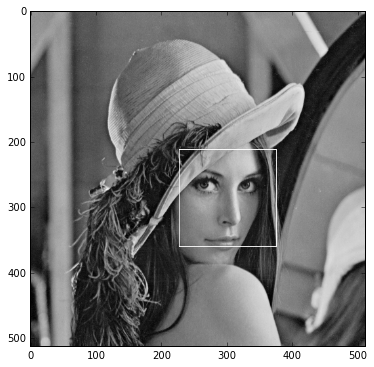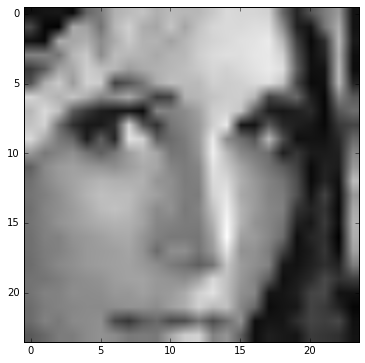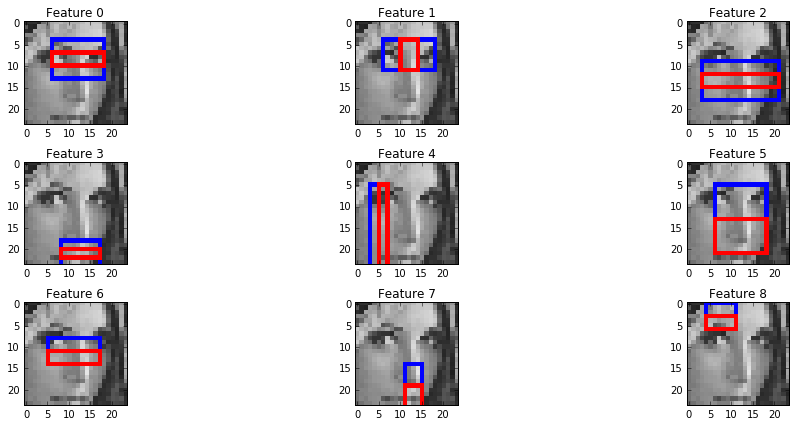In :
```%matplotlib inline
from pylab import *
rcParams['figure.figsize'] = 16, 6
import numpy as np
```

# Face Detection¶

Applying Viola-Jones based face detection in OpenCV is very simple. All you need is:

1. Obtain a trained face detection cascade
2. Apply cascade in various scales

The complete OpenCV installation comes with some very useful cascades: https://github.com/Itseez/opencv/tree/2.4.12.3/data/haarcascades Some of these cascades are for frontal face. In addition there are frontal face detection cascades that use LBP features. The cascade we will use here is: haarcascade_frontalface_default.xml

The cascade is an XML file that describes the weak classifiers learned parameters, and the HAAR features used. We will soon inspect its contents, but first lets use it.

In :
```import cv2
minSize=(64,64), # Smallest window
maxSize=(180, 180), # Largest window
scaleFactor=1.1, # Step between windoes
minNeighbors=1) # how many neighbors each candidate rectangle should have
print 'found %d faces' % len(rects)

for rect in rects:
cv2.rectangle(lena, (rect, rect), (rect+rect, rect+rect), 255)

imshow(lena); gray();
```
```found 1 faces
```In :
```lena_face_img = cv2.resize(lena[rect:(rect+rect), rect:(rect+rect)], dsize=(24,24), interpolation=cv2.INTER_CUBIC)
imshow(lena_face_img);
```## Live face detection¶

In :
```video_capture = cv2.VideoCapture(0)
while True:
if not state:
# 'Video is over'
break
minSize=(64,64), # Smallest window
maxSize=(180, 180), # Largest window
scaleFactor=1.5, # Step between windoes
minNeighbors=2) # how many neighbors each candidate rectangle should have
for rect in rects:
cv2.rectangle(img, (rect, rect), (rect+rect, rect+rect), (0, 255, 0))

cv2.imshow(winname='frame', mat=img)
key = cv2.waitKey(1) # Wait for 30 milliseconds

if key == ord('q'):
# The key 'q' has been pressed
break
cv2.destroyWindow('frame')
del video_capture
```

The cascade can be treated as a black box which magically finds faces. Luckily the cascade as represented as a somewhat readable XML file which we can inspect.

In :
```import xml.etree.ElementTree
tree = xml.etree.ElementTree.ElementTree()
features = root.find('.//features')
print 'total number of features used in cascade:', len(list(features))
print 'total stages in cascade:', len(list(root.find('.//stages')))
```
```total number of features used in cascade: 2913
```

lets examine the first stage

In :
```first_stage_weak_classifiers = list(root.find('.//stages')).find('weakClassifiers')
print 'First stage weak classifiers:', len(list(first_stage_weak_classifiers))
first_stage_features = [int(x.text.strip().split(' ')) for x in first_stage_weak_classifiers.findall('.//internalNodes')]
```
```First stage weak classifiers: 9
```

Now let's visualize the features of the first cascade by parsing the feature part of the xml:

In :
```def show_feature(feature_tag):
canvas = np.dstack([lena_face_img]*3)
for rect_tag in feature_tag.findall('.//rects/_'):
tokens = rect_tag.text.strip().split(' ')
rect = [int(x) for x in tokens[:4]]
weight = float(tokens[-1])
cv2.rectangle(canvas, tuple(rect[:2]), (rect+rect, rect+rect), color=(255,0,0) if weight > 0 else (0,0,255), thickness=1)
return canvas

for i in first_stage_features:
canvas = show_feature(features[i])
subplot(3,3,i+1)
title('Feature %d' % i)
imshow(canvas, interpolation='nearest')
tight_layout()
```